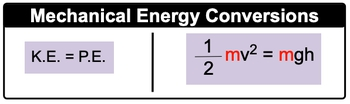## General Chemistry

Learn the toughest concepts covered in Chemistry with step-by-step video tutorials and practice problems by world-class tutors

8. Thermochemistry

# Kinetic & Potential Energy

Mechanical Energy is the energy associated with an object's velocity (Kinetic Energy) and its position (Potential Energy).

Kinetic Energy & Potential Energy
1
concept

## Kinetic & Potential Energy1m
Play a video:
kinetic energy and potential energy are connected to one another because they form part off mechanical energy. Now mechanical energy is energy and object possesses, due to its motion as kinetic energy or its position as potential energy. Now, with Connecticut and energy and potential energy, we have formulas that are important to remember. So the kinetic energy formula is used for an object in motion that has a mass and velocity which is connected to its speed. Here we say kinetic energy, which is abbreviated as K e, equals half times m times v squared here am equals the mass of the of the gas in kilograms and V equals velocity of the gas in meters per second. Kinetic energy ke e is in jewels, which is capital J, or just think about it. If this is in kilograms and were squaring meters per second, that would mean that jewels are equivalent to kilograms. Times meter squared over second squared potential energy formula is used for a stationary object that has a mass and ah, height. Now here we say, potential energy, which is abbreviated as p e equals m times g times H here, M equals the mass of the object again in kilograms. And here we're gonna say G is acceleration due to gravity. And here on earth, that is 9.8 meters over seconds squared. So that's gonna be as close as we get to physics this semester. So just keep that in mind in terms of acceleration due to gravity. For those of you are gonna take physics or have taken physics, you should know this type of idea and then h equals the height of the object in meters. So just remember, kinetic energy and potential energy are different, but they formed the sum of mechanical energy.

The kinetic energy of a gas molecule is connected to its mass in kilograms and velocity in meters per second.The potential energy of a gas molecule is connected to its mass, acceleration due to gravity and height above the ground.2
example

## Kinetic & Potential Energy Example 11m
Play a video:
here we need to calculate the kinetic energy in kill jewels of an electron which has a mass of 9.11 times 10 to the negative, 31 kg moving at 1.59 times 10 to the m per second. Remember, kinetic energy equals half times maths times velocity squared here are mass is in kilograms. So we're good times a velocity which is in meters per second. So that's also good. And then we know that we have to square this now when I square that what does it really come out? Thio? So I'm squaring everything inside of here that comes out to 2.5 to times 10 of the 40 m squared over second squared. So we have kilograms times meter squared over second squared. So that's 1.15 times 10 to the 10 kg times meter squared over second squared which remember, is the same thing as jewels. So 1.15 times 10 to the 10 jewels will be the kinetic energy of this particular electron. Here we have three sig figs in our answer because the values given to us when within the question also have three significant figures
3
Problem

A radioactive particle weighing 7.20 x 103 ng is found 110 m above the earth’s surface. What is its potential energy?

4
concept

## Kinetic & Potential Energy34s
Play a video:
now remember, Since kinetic energy and potential energy are forms of mechanical energy, you can convert between them. So that would mean that kinetic energy equals potential energy, which would mean that half times the mass of an object in kilograms times velocity squared equals the same mass of the object times gravity due to acceleration times, the height of the object and meters. So just remember, if you're given kinetic energy, there are ways to convert it into potential energy, and if you're given potential energy, you can convert it to kinetic energy.5
example

## Kinetic & Potential Energy Example 22m
Play a video: